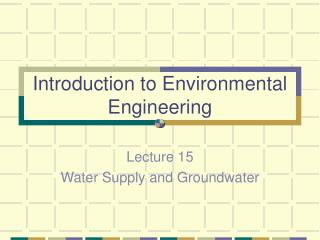DownloadDownload PresentationIntroduction to Environmental Engineering

# Introduction to Environmental Engineering

Download Presentation## Introduction to Environmental Engineering

- - - - - - - - - - - - - - - - - - - - - - - - - - - E N D - - - - - - - - - - - - - - - - - - - - - - - - - - -
##### Presentation Transcript

1. Introduction to Environmental Engineering Lecture 15 Water Supply and Groundwater

2. Water • Population Density • The key to water is balance with the environment • We aren’t in balance • The natural environment will force Balance, Equilibrium

3. Hydrologic Cycle • Cycle of Water • The basics that you already know: • Precipitation, percolation, runoff, evapotranspiration, groundwater, surface water • Sources of Drinking water • Groundwater and Surface Water • Groundwater terms • Zone of aeration, zone of saturation, water table, aquifer, confined aquifer, & artesian well

4. Groundwater supplies • Often when groundwater is usually very little if any treatment is performed before consumption. • Groundwater is polluted by point and non-point sources just like surface waters. • A major difference in the pollution of groundwater and the pollution of surface waters is that groundwater is usually used by an individual consumer that can not afford the “multiple barrier concept” of treatment.

5. Multiple Barrier Concept • 1.Effective Natural Barriers, Improve and Protect Raw Water Quality, and Effective sewage treatment upstream of the source • 2.Removal of Microbes – Coagulation, Flocculation, Sedimentation, Sand Filtration • 3.Inactivation of microbes by Chlorine disinfection • Any one barrier is fallible, Full system is a “fail safe” approach

6. Groundwater Flow • Groundwater moves through different soils at different rates based on the experimentally determined coefficient of permeability, K • K as high as 10 m/s – 10–14 m/s • Models are available for the subsurface that are simple and complex • Very hard to predict because of the heterogeneity of most aquifers

7. Porosity and Specific Yield • The amount of water that can be stored in an aquifer is equal to the volume of space between the soil grains • Porosity

8. Specific Yield • All water is not available for extraction

9. Darcy’s Law for Groundwater flow

10. Darcy’s Law • Q = flow (horizontal) through the aquifer, m3/s • K = coefficient of permeability, m/s • A = cross-sectional area, m2 • h2 – h1 = head drop, m • l2 –l1 = length difference, m

11. Specific Discharge • v’ = Av/a = Q/a • v’ = specific discharge, or flow per unit area, m/s • If porosity is known pore velocity can be calculated by: • v/porosity = actual water velocity • To calculate time from point of pollution to a down gradient point at a known distance. • This can be combined with a first or zero order rate of decay.

12. Example • If the water wells spaced at 5 km in a 20 m thick confined aquifer are used to monitor groundwater quality and the upstream well detects a pollutant of concentration 100 mg/L. The head loss between the two wells is 10 m/m. Assuming a decay coefficient of k = 10-4 day-1 and a coefficient of permeability of 10-3 m/s, determine the concentration in the downstream well.

13. Solution • First calculate the velocity of the groundwater, to determine how many days it will take to reach the downstream well. • Gradient (head loss)= 10 m/m • K = hydraulic conductivity = 1 x 10-3 m/s • k = first order decay rate = 1 x10-4 day-1 • V = Darcy velocity = K dh/dL = 1 x 10-3 (10 m/5000m) = 2 x 10-6 m/s • t = x/v = 5000m/2 x10-6 m/s = 2.5 x109s x 1hr/3600s x 1 day/24 hr = 28,935 days • Well concentration = Ct = Co e-kt = 100 mg/L e-(1x10-4days-1)(28,935 days) = 5.53 mg/L